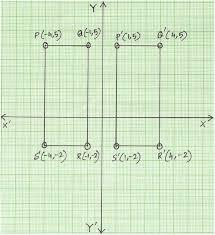# Circumference 6040

The picture shows a square net in which the side of one square is 1 cm long. Draw a rectangle with an area of 18 squares with a circumference of 22 cm.

a =  9 cm
b =  2 cm

### Step-by-step explanation:Did you find an error or inaccuracy? Feel free to write us. Thank you!

Tips for related online calculators
Are you looking for help with calculating roots of a quadratic equation?
Do you have a system of equations and looking for calculator system of linear equations?

#### You need to know the following knowledge to solve this word math problem:

We encourage you to watch this tutorial video on this math problem: